# Combine text from multiple cells into one cell in Excel

In this article, we will show you how to combine text from multiple cells into one cell in Excel. Combining text from multiple cells into one cell in Excel is very useful for users like users who want to create a mailing list, prepare data for import, etc.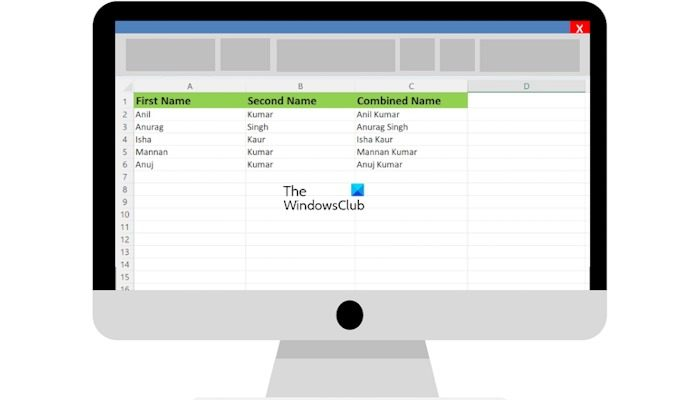## Combine text from multiple cells into one cell in Excel

There are two methods to combine text from multiple cells into one cell in Excel. Use the following steps to combine text from multiple cells into one cell in Excel.

1. By using the Ampersand symbol (&)
2. By using the CONCATENATE function

Let’s start.

#### 1] By using the Ampersand symbol (&)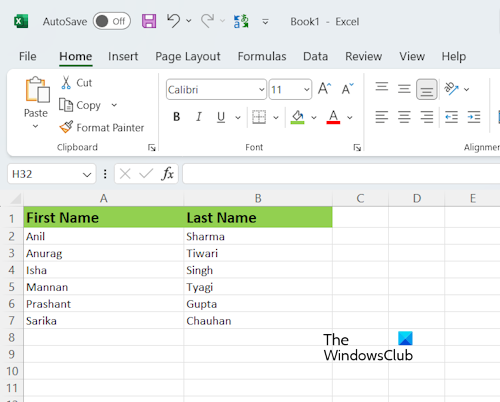The ampersand symbol is a quick and easy way to combine data in Excel. To explain this method to you, I have created sample data in Excel with the names of some persons (refer to the above screenshot). I am going to combine the first and last names of persons in my Excel worksheet.

The structure of the formula is as follows:

`=First Name&""&Second Name`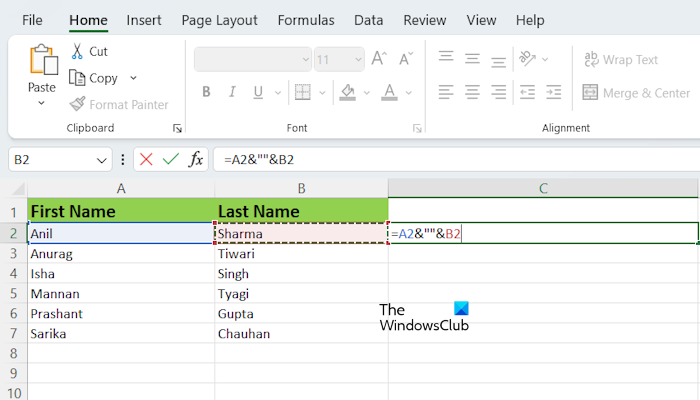I have applied the same formula to my Excel sheet. Replace the First Name and Second Name with the exact cell numbers in your Excel sheet as I did. In my case, I applied the formula to combine the names in cells A2 and B2, hence the formula looks like this:

`=A2&""&B2`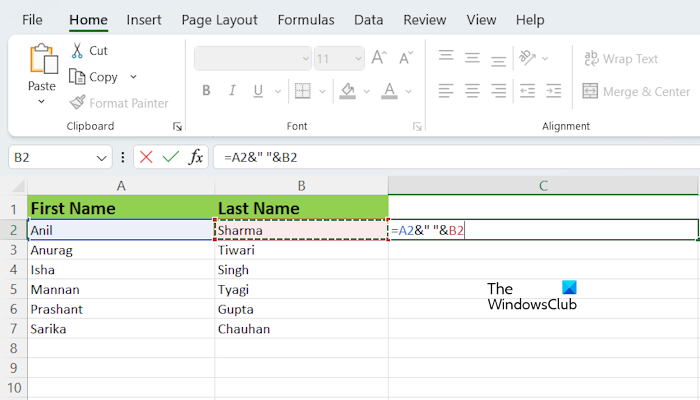The above formula combines the names without space in between them. If you want a space between the combined names, you have to add space between the quotation marks or inverted commas in the formula. Hence, in my case, the formula will become:

`=A2&" "&B2`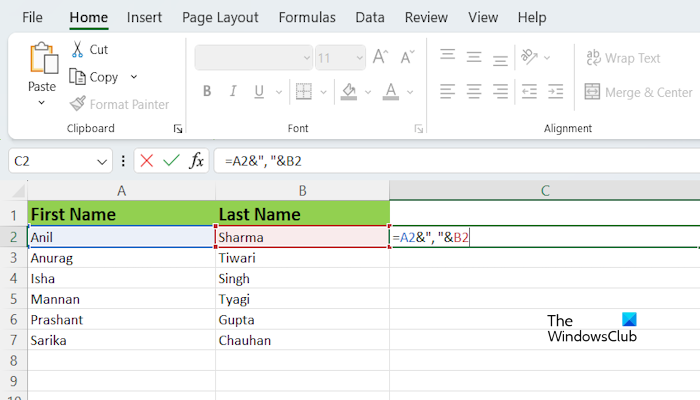If you want to insert a special character between the combined names, you have to write it between the quotation marks. For example, if you want to combine names with comma between them, the formula will look like:

`=A2&","&B2`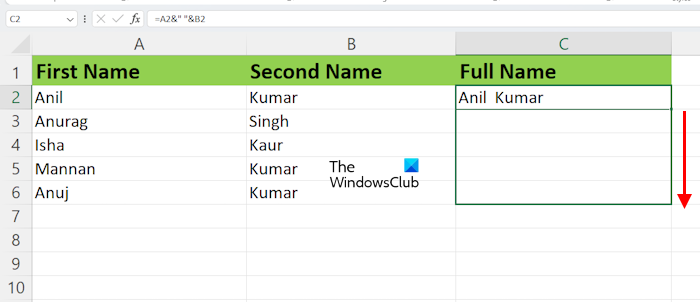After typing the formula in the targeted cell, hit Enter. You will see the result. Not, you have to apply the same formula to all the cells. To do so, you can use the Fill Handle in Excel. Hover your mouse cursor over the bottom right side of the targeted cell (say, C in my case). The cursor should be turned into a black plus icon. Now, press and hold the left button of your mouse, drag it to the bottom, and then release the left mouse click.

#### Combining more than two names by using the Ampersand symbol

If you want to combine more than two names with the help of the Ampersand symbol, the structure of the formula will look like this: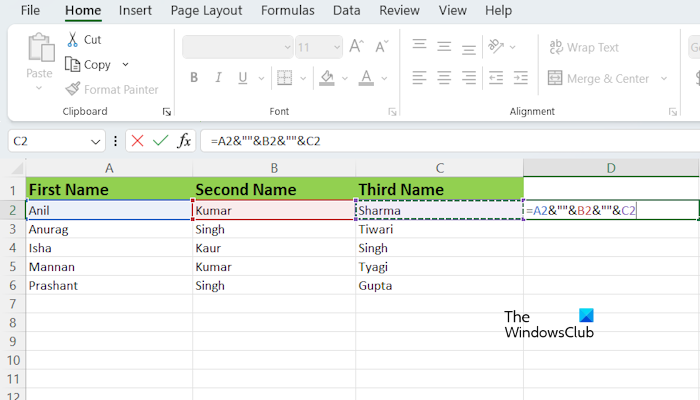`=First Name&""&Second Name&""&Third Name`

You have to add the Ampersand symbol before and after all the middle names. For example, if there are four names, the formula will become:

`=First Name&""&Second Name&""&Third Name&""&Fourth Name`

If you want to add space between names, separate the inverted commas in the formula with space. Now, apply the same formula to all the remaining cells by using the Fill Handle.

#### 2] By using the CONCATENATE functionThe CONCATENATE function is a text function in Excel that allows you to combine more than two strings together into one string. CONCATENATE is a quick and easy way to combine data in Excel.

The structure of the formula is as follows:

`=CONCATENATE(First Name, Second Name)`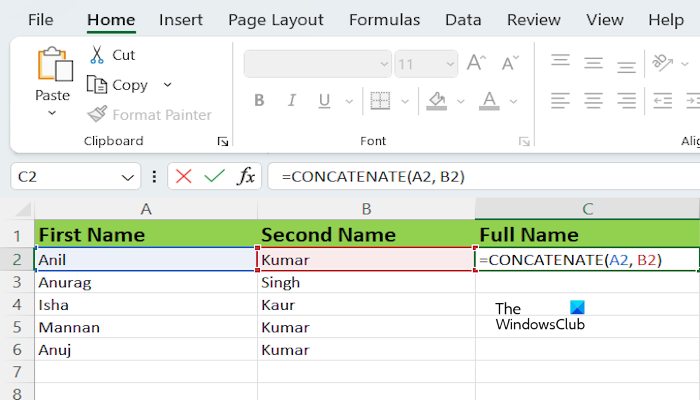Do not forget to replace the first name and the second name with the exact cell numbers in your Excel sheet. In my case, the formula looks like this:

`=CONCATENATE(A2, B2)`

#### Combining more than two names by using the CONCATENATE Function

If you want to combine more than two names with the help of the CONCATENATE Function, the structure of the formula will look like this: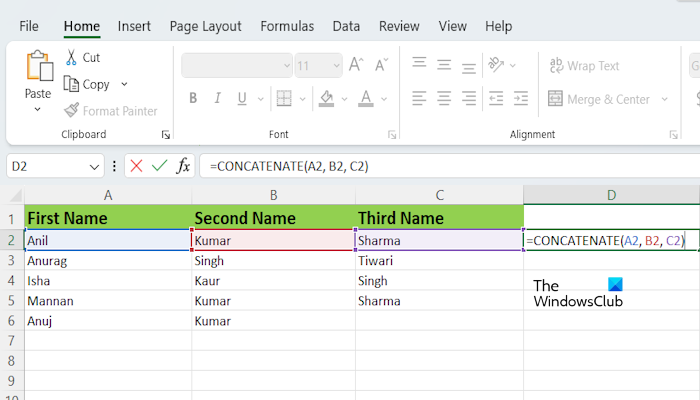`=CONCATENATE(text1, text2, ..., text255)`

You can combine text up to 255 text arguments by using the CONCATENATE Function. Each can be a string or array of strings, such as a range of cells.

In Microsoft Office 365 and Microsoft Office versions 2019 and later, Microsoft has replaced the CONCATENATE function with CONCAT. However, the CONCATENATE function still works. If you are using the newer version of Microsoft Office, you can replace CONCATENATE in the above formula with the CONCAT function (if the CONCATENATE function does not work for you). Everything else will remain the same.

That’s it. I hope this article helps to Combine text from multiple cells into one cell in Excel.

Read: How to create a Formula to Add, Subtract, Multiply, or Divide in Excel.

### How do I combine 3 text cells into one?

You can use the CONCATENATE or CONCAT function to combine the text in 3 cells into one cell in Excel. The Ampersand symbol also does the same job but it becomes a bit difficult to use when it comes to more than 2 texts in Excel.

### How do I merge cells in Excel and keep all text?

It is not possible to keep all the text in a merged cell. If all the cells contain some values and you merge all of them, then Excel will take only the first value and delete all the other values after merging the cells.#Combine #text #multiple #cells #cell #Excel